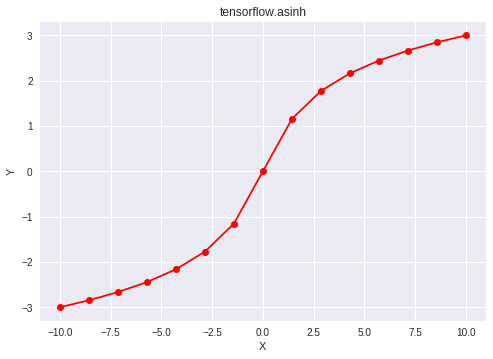# Python | Tensorflow asinh() method

Tensorflow is an open-source machine learning library developed by Google. One of its applications is to develop deep neural networks.

The module `tensorflow.math` provides support for many basic mathematical operations. Function `tf.asinh()` [alias `tf.math.asinh`] provides support for the inverse hyperbolic sine function in Tensorflow. The input type is tensor and if the input contains more than one element, element-wise inverse hyperbolic sine is computed.

Syntax: tf.asinh(x, name=None) or tf.math.asinh(x, name=None)

Parameters:
x: A tensor of any of the following types: float16, float32, float64, complex64, or complex128.
name (optional): The name for the operation.

Return type: A tensor with the same type as that of x.

Code #1:

 `# Importing the Tensorflow library ` `import` `tensorflow as tf ` `   `  `# A constant vector of size 6 ` `a ``=` `tf.constant([``1.0``, ``-``0.5``, ``3.4``, ``22.1``, ``0.0``, ``-``6.5``], ` `                               ``dtype ``=` `tf.float32) ` `   `  `# Applying the asinh function and ` `# storing the result in 'b' ` `b ``=` `tf.asinh(a, name ``=``'asinh'``) ` `   `  `# Initiating a Tensorflow session ` `with tf.Session() as sess: ` `    ``print``(``'Input type:'``, a) ` `    ``print``(``'Input:'``, sess.run(a)) ` `    ``print``(``'Return type:'``, b) ` `    ``print``(``'Output:'``, sess.run(b)) `

Output:

```Input type: Tensor("Const_1:0", shape=(6, ), dtype=float32)
Input: [ 1.  -0.5  3.4 22.1  0.  -6.5]
Return type: Tensor("asinh:0", shape=(6, ), dtype=float32)
Output: [ 0.8813736  -0.48121184  1.9378793   3.7892363   0.         -2.5708146 ]
```

Code #2: Visualization

 `# Importing the Tensorflow library ` `import` `tensorflow as tf ` `  `  `# Importing the NumPy library ` `import` `numpy as np ` `  `  `# Importing the matplotlib.pylot function ` `import` `matplotlib.pyplot as plt ` `  `  `# A vector of size 15 with values from -10 to 10 ` `a ``=` `np.linspace(``-``10``, ``10``, ``15``) ` `  `  `# Applying the inverse hyperbolic sine ` `# function and storing the result in 'b' ` `b ``=` `tf.asinh(a, name ``=``'asinh'``) ` `  `  `# Initiating a Tensorflow session ` `with tf.Session() as sess: ` `    ``print``(``'Input:'``, a) ` `    ``print``(``'Output:'``, sess.run(b)) ` `    ``plt.plot(a, sess.run(b), color ``=` `'red'``, marker ``=` `"o"``)  ` `    ``plt.title(``"tensorflow.asinh"``)  ` `    ``plt.xlabel(``"X"``)  ` `    ``plt.ylabel(``"Y"``)  ` `  `  `    ``plt.show() `

Output:

```Input: [-10.          -8.57142857  -7.14285714  -5.71428571  -4.28571429
-2.85714286  -1.42857143   0.           1.42857143   2.85714286
4.28571429   5.71428571   7.14285714   8.57142857  10.        ]
Output: [-2.99822295 -2.84496713 -2.66412441 -2.44368627 -2.16177575 -1.77227614
-1.15447739  0.          1.15447739  1.77227614  2.16177575  2.44368627
2.66412441  2.84496713  2.99822295]
```My Personal Notes arrow_drop_upCheck out this Author's contributed articles.

If you like GeeksforGeeks and would like to contribute, you can also write an article using contribute.geeksforgeeks.org or mail your article to contribute@geeksforgeeks.org. See your article appearing on the GeeksforGeeks main page and help other Geeks.

Please Improve this article if you find anything incorrect by clicking on the "Improve Article" button below.

Article Tags :

Be the First to upvote.

Please write to us at contribute@geeksforgeeks.org to report any issue with the above content.